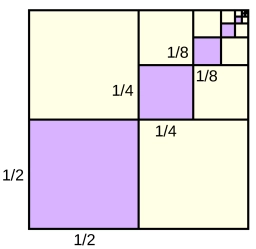# In which

In which of the following expressions does the number 16 fill in the blank so that the equation is true? Select all that apply.

A) 8(___ + 3) = 32 + 24
B) 8(2 + 9) = ___ + 72
C) 4(7 + 4) = 28 + ___
D) 8(5 + 6) = 40 + ___

Result

A=

B=

C=

D=

### Step-by-step explanation:Did you find an error or inaccuracy? Feel free to write us. Thank you!

Tips for related online calculators
Do you have a linear equation or system of equations and looking for its solution? Or do you have a quadratic equation?